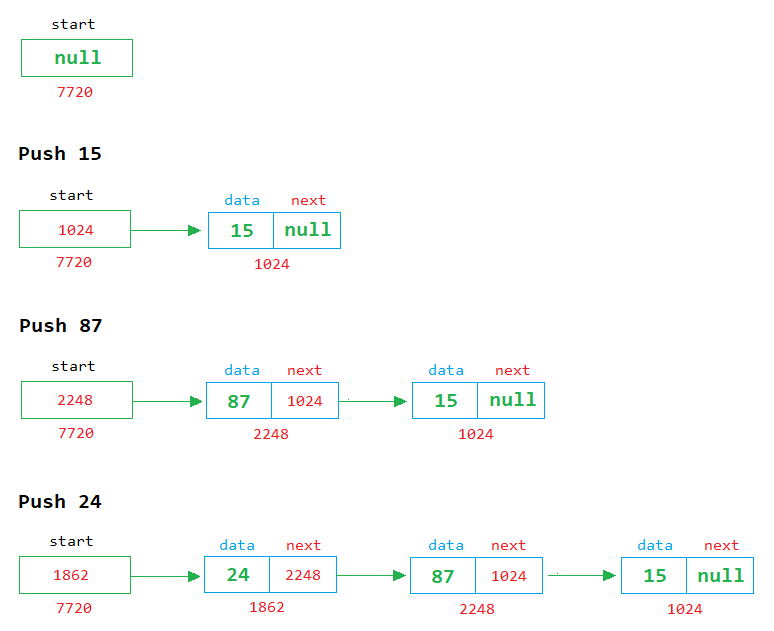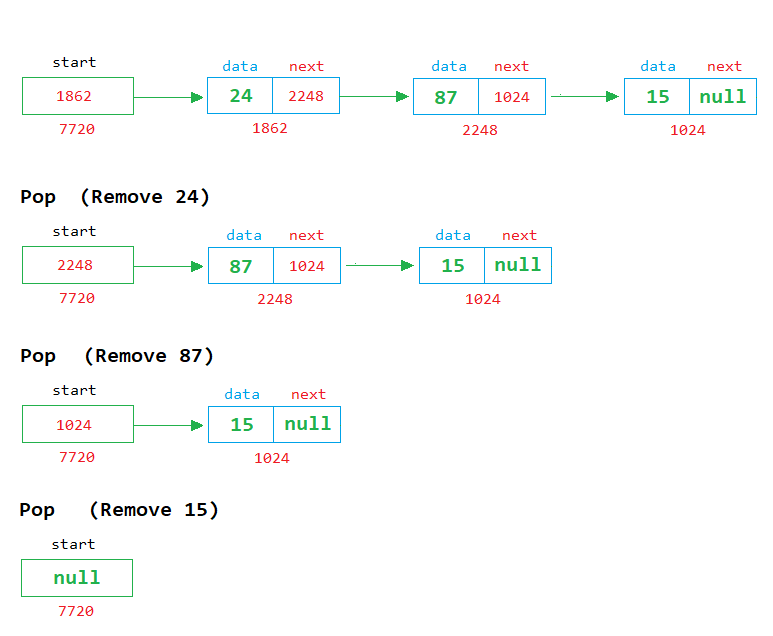# Stack using Linked List in Java

###### Data Structures in Java

In this lesson, we will learn how to implement the stack using a singly linked list in java.

## Linked List Implementation of Stack in Java

We know about the stack and how to implement it using an array. In this lesson, we will learn how to implement the stack using a singly linked list.

We also know that two operations on the stack are possible: push and pop. See the image below to understand how to implement push and pop operations on a stack using a linked list.

### Push ExampleIn the above image, we can see that when a new node is created, it is placed in front of the first node, and its address is stored in the start variable.

### Pop ExampleIn the above image, we can see that each time a node is removed, the first node is deleted from the list, and the address of the next node is stored in the start variable.## Program of Stack using Linked List

Below is the complete program of stack in java using singly linked list having size 5.

#### Example

``````import java.util.Scanner;

// define Node class
class Node
{
public int data = 0;
public Node next = null;
}

{
public static void main(String args[])
{
Node start=null, temp;
int top=0, ch, n, size=5;
Scanner sc=new Scanner(System.in);

for(;;)		// An infinite loop
{
System.out.println("\n1. Push");
System.out.println("2. Pop");
System.out.println("3. Display");
System.out.println("4. Exit");
System.out.print("Enter Choice: ");
ch=sc.nextInt();

switch(ch)
{
case 1:
if(top==size)
{
System.out.println("Stack is full");
}
else
{
// Enter a number to store in node
System.out.print("Enter a number ");
n=sc.nextInt();

// create a node object in temp
temp=new Node();
temp.data=n;		// assign the value of n in the data part of temp
temp.next=null;		// assign the null in the next part of temp

if(start==null)		// if start in null
{
start=temp;		// then assing the temp in start (start points to the first node in the memory)
}
else
{
// insert the new node before the first node
temp.next=start;
start=temp;
}
top++;
}
break;

case 2:
if(start==null)
{
System.out.println("Stack is empty");
}
else
{
System.out.println("Number Popped = " + start.data);
temp=start;
start=start.next;
temp=null;		// remove the reference of temp node
top--;
}
break;

case 3:
if(start==null)
{
System.out.println("Stack is empty");
}
else
{
temp=start;		// start from 1st node

// display the nodes on the screen
while(temp!=null)
{
System.out.println(temp.data);
temp=temp.next;
}
}
break;

case 4:
System.exit(0);
break;

default:
System.out.println("Wrong Choice");
}
}
}
}``````